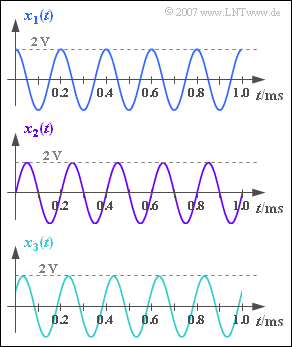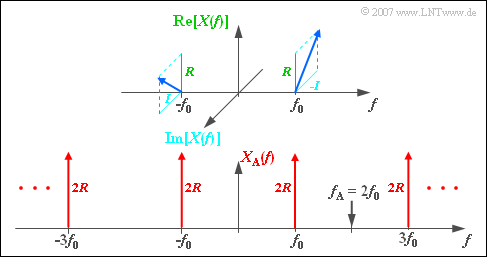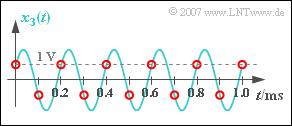# Exercise 5.1Z: Sampling of Harmonic OscillationsThree harmonic oscillations of equal frequency  $f_0$  and equal amplitude  $A$

We consider three harmonic oscillations with the same frequency and the same amplitude:

$$x_1(t) = A \cdot \cos (2 \pi \cdot f_0 \cdot t) \hspace{0.05cm},$$
$$x_2(t) = A \cdot \sin (2 \pi \cdot f_0 \cdot t) \hspace{0.05cm},$$
$$x_3(t) = A \cdot \cos (2 \pi \cdot f_0 \cdot t - 60^{\circ}) \hspace{0.05cm}.$$

The oscillation parameters  $f_0$  and  $A$  can be taken from the graph.

It is assumed that the signals are sampled equidistantly at times  $\nu \cdot T_{\rm A}$  whereby the parameter values  $T_{\rm A} = 80 \ µ \text{s}$  and  $T_{\rm A} = 100 \ µ \text{s}$  are to be analysed.

The signal reconstruction at the receiver takes place through a low-pass filter  $H(f)$,  which forms the signal  $y_{\rm A}(t) = x_{\rm A}(t)$  from the sampled signal  $y(t)$ . It applies:

$$H(f) = \left\{ \begin{array}{c} 1 \\ 0.5 \\ 0 \\ \end{array} \right.\quad \begin{array}{*{5}c} {\rm{{\rm{f\ddot{u}r}}}} \\ {\rm{{\rm{f\ddot{u}r}}}} \\ {\rm{{\rm{f\ddot{u}r}}}} \\ \end{array}\begin{array}{*{5}c} |f| < f_{\rm G} \hspace{0.05cm}, \\ |f| = f_{\rm G} \hspace{0.05cm}, \\ |f| > f_{\rm G} \hspace{0.05cm}, \\ \end{array}$$

Here  $f_{\rm G}$  indicates the cut-off frequency of the rectangular low-pass filter.  For this shall apply:

$$f_{\rm G} = \frac{1}{ 2 \cdot T_{\rm A}}\hspace{0.05cm}.$$

The sampling theorem is fulfilled if  $y(t) = x(t)$  holds.

Hints:

### Questions

1

What are the amplitude and frequency of the signals  $x_1(t)$,  $x_2(t)$  and  $x_3(t)$?

 $A \hspace{0.25cm} = \$  $\text{V}$ $f_0\hspace{0.2cm} = \$  $\text{kHz}$

2

For which input signals is the sampling theorem satisfied   ⇒   $y(t) = x(t)$, when  $\underline{T_{\rm A} = 80 \ {\rm µ} \text{s}}$ ?

 $x_1(t)$, $x_2(t)$, $x_3(t)$.

3

What is the reconstructed signal  $y_1(t) = A_1 \cdot \cos (2\pi f_0 t – \varphi_1)$  with the sampling distance  $\underline{T_{\rm A} = 100 \ {\rm µ} \text{s}}$? Interpret the result.

 $A_1\hspace{0.2cm} = \$  $\text{V}$ $\varphi_1\hspace{0.2cm} = \$  $\text{Deg}$

4

What is the amplitude  $A_2$  of the reconstructed signal  $y_2(t)$, when the sinusoidal signal  $x_2(t)$  is present?  Let  $\underline{T_{\rm A} = 100 \ {\rm µ} \text{s}}$ still apply.

 $A_2\hspace{0.2cm} = \$  $\text{V}$

5

What is the amplitude  $A_3$  of the reconstructed signal  $y_3(t)$, when the signal  $x_3(t)$  is present?   $\underline{T_{\rm A} = 100 \ {\rm µ} \text{s}}$ is still valid.

 $A_3\hspace{0.2cm} = \$  $\text{V}$

### Solution

#### Solution

(1)  The graph shows the amplitude  $\underline{A = 2\ \text{V}}$  and the period  $T_0 = 0.2 \ \text{ms}$.

• This results in the signal frequency  $f_0 = 1/T_0 \; \underline{= 5 \ \text{kHz}}$.

(2)  All proposed solutions are correct:

• The sampling rate here is  $f_{\rm A} = 1/T_{\rm A} = 12.5 \ \text{kHz}$.
• This value is greater than  $2 \cdot f_0 = 10 \ \text{kHz}$.
• Thus the sampling theorem is fulfilled independently of the phase and  $y(t) = x(t)$ always applies.Spectrum  $X_{\rm A}(f)$  of the sampled signal – real part and imaginary part

(3)  The sampling rate is now  $f_{\rm A} = 2 \cdot f_0 = 10 \ \text{kHz}$.

• Only in the special case of the cosine signal the sampling theorem is satisfied, and it holds:
$$y_1(t) = x_1(t) ⇒ A_1 \; \underline{=2 \ \text{V}} \text{ und }\varphi_1 \; \underline{= 0}.$$

This result is now to be derived mathematically, whereby a phase  $\varphi$  in the input signal is already taken into account with regard to the remaining subtasks:

$$x(t) = A \cdot \cos (2 \pi \cdot f_0 \cdot t - \varphi) \hspace{0.05cm}.$$
• Then, for the spectral function sketched in the graph above:
$$X(f) = {A}/{2} \hspace{0.05cm} \cdot \hspace{0.05cm} {\rm e}^{{\rm j} \hspace{0.05cm} \cdot \hspace{0.05cm} \varphi} \cdot \delta (f+ f_{\rm 0} ) + {A}/{2} \hspace{0.05cm} \cdot \hspace{0.05cm} {\rm e}^{-{\rm j} \hspace{0.05cm} \cdot \hspace{0.05cm} \varphi} \cdot \delta (f- f_{\rm 0} )\hspace{0.05cm}.$$
• With the abbreviations
$$R = {A}/{2} \hspace{0.05cm} \cdot \hspace{0.05cm} \cos(\varphi) \hspace{0.5cm}{\rm and} \hspace{0.5cm}I ={A}/{2} \hspace{0.05cm} \cdot \hspace{0.05cm} \sin(\varphi)$$
can also be written for this:
$$X(f) = (R + {\rm j} \cdot I) \cdot \delta (f+ f_{\rm 0} ) + (R - {\rm j} \cdot I) \cdot \delta (f- f_{\rm 0} )\hspace{0.05cm}.$$
• The spectrum of the signal  $x_{\rm A}(t)$  sampled with  $f_{\rm A} = 2f_0$  is thus:
$$X_{\rm A}(f) = \sum_{\mu = - \infty }^{+\infty} X (f- \mu \cdot f_{\rm A} )= \sum_{\mu = - \infty }^{+\infty} X (f- 2\mu \cdot f_{\rm 0} )\hspace{0.05cm}.$$
• The bottom graph shows that  $X_{\rm A}(f)$  consists of Dirac functions at  $\pm f_0$,  $\pm 3f_0$,  $\pm 5f_0$,  and so on.
• All weights are purely real and equal to  $2 \cdot R$.
• The imaginary parts of the periodically continued spectrum cancel out.
• If one further takes into account the rectangular low-pass filter whose cut-off frequency lies exactly at  $f_{\rm G} = f_0$,  as well as   $H(f_{\rm G}) = 0.5$, one obtains for the spectrum after signal reconstruction:
$$Y(f) = R \cdot \delta (f+ f_{\rm 0} ) + R \cdot \delta (f- f_{\rm 0} )\hspace{0.05cm}, \hspace{0.5cm} R = {A}/{2} \hspace{0.05cm} \cdot \hspace{0.05cm} \cos(\varphi)\hspace{0.05cm}.$$
• The inverse Fourier transformation leads to
$$y(t) = A \cdot \cos (\varphi)\cdot \cos (2 \pi \cdot f_0 \cdot t ) \hspace{0.05cm}.$$
• Thus, a cosine-shaped progression results independent of the input phase  $\varphi$ .
• If  $\varphi = 0$  as with the signal  $x_1(t)$, the amplitude of the output signal is also equal to  $A$.

(4)  The sine signal has the phase  $90^\circ$.

• From this follows directly  $y_2(t) = 0$   ⇒   amplitude $\underline{A_2 = 0}$.
• This result becomes understandable if you look at the samples in the graph.
• All samples (red circles) are zero, so there can be no signal even after the filter.Reconstruction of a harmonic oscillation with  $60^\circ$ phase

(5)  Despite   ⇒   $\varphi = 60^\circ$ gilt $\varphi_3 = 0$   ⇒   the reconstructed signal  $y_3(t)$  is cosine-shaped, too.

• The amplitude is equal to
$$A_3 = A \cdot \cos (60^{\circ})= {A}/{2} \hspace{0.15 cm}\underline{= 1\,{\rm V}} \hspace{0.05cm}.$$
• If you look at the samples drawn in red in the graph, you will admit that you as a "signal reconstructor" would not make any other decision than the low-pass.
• Because, you don't know the turquoise curve.